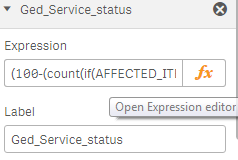# QlikView Layout & Visualizations

Discussion Board for collaboration on QlikView Layout & Visualizations.

Highlighted
New Contributor II

## Re: Qlik sense desktop manage colours in expression panel

Hi Oleg,

thank you so much for information. I receive always an error in expression panel I suppose for ">= 0.80 and <= 0.90" .

I need to use a "between" formula.

Regards

DanieleMVP

## Re: Qlik sense desktop manage colours in expression panel

Indeed, you cannot implicitly recover part of an expression during evaluation (the expression evaluator itself may decide to do so, but you cannot shorten the syntax). You'll have to write the two parts in their entirety, like:

:

if(

(count({< AFFECTED_ITEM={'Ged'}, STATUS={'open'} >}  NUMBER)*0.01-1 >= 0.80) and

(count({< AFFECTED_ITEM={'Ged'}, STATUS={'open'} >}  NUMBER)*0.01-1 <= 0.90),

rgb(220,181,43),

rgb(169,54,41)

)

:MVP & Luminary

## Re: Qlik sense desktop manage colours in expression panel

If the calculation involves one of the chart measures, you should be able to refer to them by name, though...

New Contributor II

## Re: Qlik sense desktop manage colours in expression panel

Hi Oleg,

the last conversation is very intersting...

This is the situation:

1) last configuration in expression panel is OK but not worked, change colour isnt' good when threshold is in line.

2) measure label is named "Ged_Service_status" and the value obtained is expressed as a percentage

At this point, things start to become fascinating

Daniele

New Contributor II

## Re: Qlik sense desktop manage colours in expression panel

this is the formula in expression to obtain the value:

(100-(count(if(AFFECTED_ITEM='Ged' and STATUS='open',NUMBER))))/100

and in value color formula is:

if(

100-(count(if(AFFECTED_ITEM='Ged' and STATUS='open',NUMBER)))/100 >= 0.10 and

100-(count(if(AFFECTED_ITEM='Ged' and STATUS='open',NUMBER)))/100 <= 0.95,

rgb(169,54,41),

rgb(78,133,65)

)

Regards

DanieleMVP & Luminary

## Re: Qlik sense desktop manage colours in expression panel

Hi Daniele,

I'm not sure I understand the problem anymore... it would be a lot easier to troubleshoot if you could post an example of your app and the formula that you'd like to improve.

New Contributor II

## Re: Qlik sense desktop manage colours in expression panel(100-(count(if(AFFECTED_ITEM='Ged' and STATUS='open',NUMBER))))/100 (output is "6 Incidents")if(

100-(count(if(AFFECTED_ITEM='Ged' and STATUS='open',NUMBER)))/100 >= 0.10 and

100-(count(if(AFFECTED_ITEM='Ged' and STATUS='open',NUMBER)))/100 <= 0.95,

rgb(169,54,41),

rgb(78,133,65)

)

Output is "94 %".

I was expecting to obtain a red colour because % is between 10 and 95 %.

I hope it seems more understandable.

What do you think about ?

Regards

Daniele

New Contributor II

## Re: Qlik sense desktop manage colours in expression panel(100-(count(if(AFFECTED_ITEM='Ged' and STATUS='open',NUMBER))))/100 (output is "6 Incidents")if(

100-(count(if(AFFECTED_ITEM='Ged' and STATUS='open',NUMBER)))/100 >= 0.10 and

100-(count(if(AFFECTED_ITEM='Ged' and STATUS='open',NUMBER)))/100 <= 0.95,

rgb(169,54,41),

rgb(78,133,65)

)

Output is "94 %".

I was expecting to obtain a red colour because % is between 10 and 95 %.

I hope it seems more understandable.

What do you think about ?

Regards

Daniele